# EE308FZ Lab1-2

The Link of Requirement of This Assignmenthttps://bbs.csdn.net/topics/608734907
The Aim of This AssignmentLab1_2_solution
MU STU ID and FZU STU IDMU:20124732_FZU:832001124

-> My Github link of this project: https://github.com/fish-Rita/EE308FZ-Lab1-2

Part1: PSP form

Part2: Description of problem-solving ideas

Part3: Design and implementation process

- level 1

- level 2

- level 3 & 4

Part4: Code description

Part5: Unit test

Input level = 1 :

Input level = 2 :

Input level = 3 :

Input level = 4 :

Part6: Coverage optimization & Test performance

1.coverage optimization

2.Test performance

Part7: Summary

# Part1: PSP form

Personal Software Process StagesEstimated Time/minutesCompleted Time/minutes
Planning3030
Estimate2025
Development7530
Analysis9050
Design Spec6540
Design Review3030
Coding Standard2530
Design3030
Coding4890
Code Review Planning4040
Test6025
Reporting3540
Test Report7080
Size Measurement3040
Postmortem&Process Improvement3030

# Part2: Description of problem-solving ideas

At the beginning, I decided to use C++ to solve the Lab as I am more skillful at C++. After understanding the requirements of the problem, I started to think about a good general idea: The topic roughly requires us to complete 4 levels of tasks.

First of all, we must input preprocess files, and then complete the realization of Lab functions. Once we have established this idea,  we can start writing code.

# Part3: Design and implementation process

My thoughts about the organiztion and stucture of codes is show below by using 4 pictures of mind mappings

### - level 1

We need to input the sample files, use substr to cut on the string and pushback it to the vector as the course of preprocessing the strings, then I compare this string we interested in with the keywords using interation, if it is, then do incresement.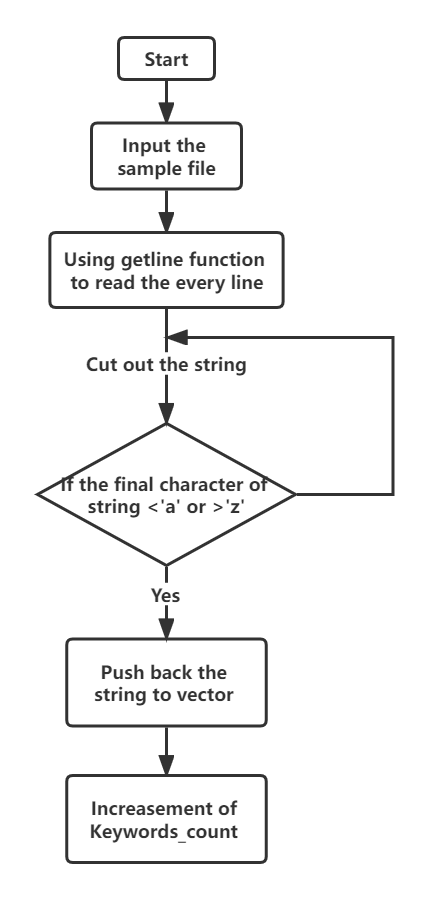### - level 2

Iterate to find the switch and case until the next switch or end of stored vector, do the increasement. After gaining the number of "switch" structures, I use a loop to find the number of  case until the next switch or completion of iteration.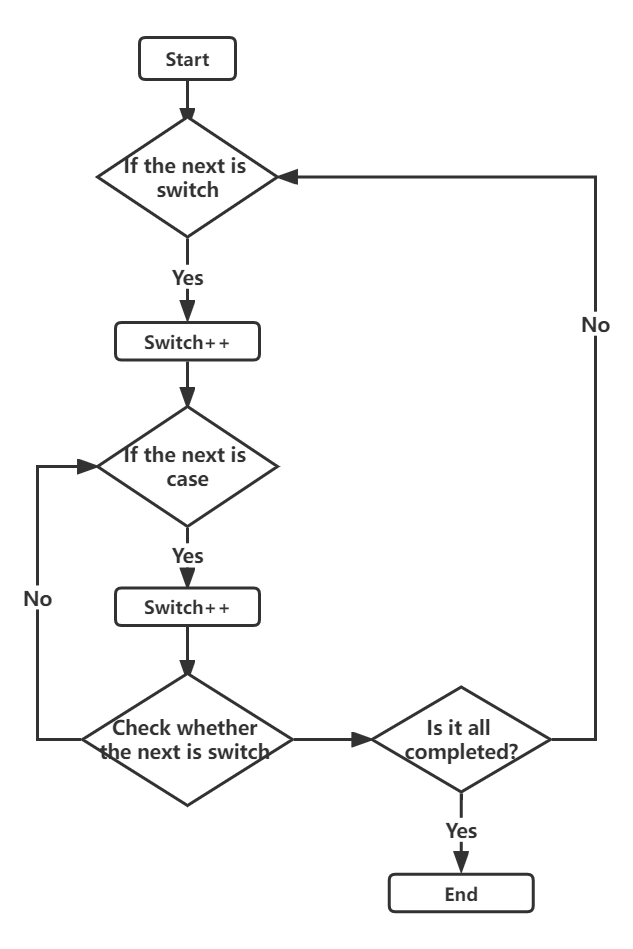### - level 3 & 4

Count the total num of if ,else,else if, and check if inside if, then check whether the next is else or the end of  completion, during that I set some flags, then calculate the final results.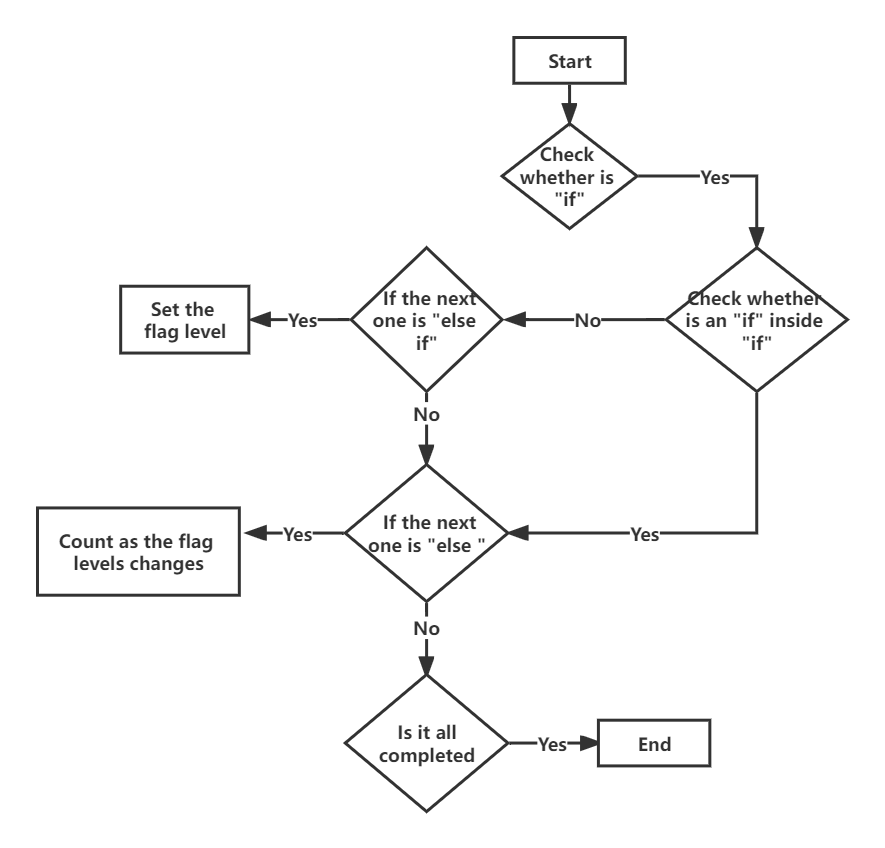# Part4: Code description

``````#include<iostream>
#include<fstream>
#include<vector>
using namespace std;
int main()
{
string text;
vector<string> nums;
string filename;
string keywords={"auto", "break", "case", "char", "const", "continue",
"default", "do", "double", "else", "enum", "extern", "float",
"for", "foto", "if", "int", "long", "main", "register", "return", "short",
"signed", "sizeof", "static", "struct", "switch", "typeof", "union",
"unsigned", "void", "volatile", "while"};
string ifelsekeywords={"else if","if","else"};
int keywdcnt = {0}, ifelsekeywdcnt = {0},keyword_count=0,switch_count=0,case_count=0,elseifcount=0;
vector<int> casenum;
vector<string> ifelsearr;
int ifelsecount=0, ifelseifcount=0, if_num=0, elseifnum=0, else_num=0;``````

choose the level and open the file

``````ifstream inFile;
cout <<"Please choose the level(1,2,3,4): ";
int level;
cin>>level;
cout << "Please input the file path:";
cin >> filename;
inFile.open(filename);  //open the file sample.cpp
if(inFile.fail())
{
cout<<"The file was not successfully opend"<<endl;
return 0;
}``````

(1) level 1: count the total num of keywords

``````	//level 1
while (getline(inFile,buf))
{
cout << buf << endl;
int i = 0, j = 0;
while(i < int(buf.size()) && j < int(buf.size()))
{
if (buf[i] < 'z' && buf[i] > 'a')
{
if (buf[j] > 'z' || buf[j] < 'a')
{
string s = buf.substr(i, j-i);
nums.push_back(s);
for (int k = 0; k < 33; k++) {
if (s == keywords[k]) {
keyword_count++;
//	keywdcnt[k]+=1;
}
}
i = j+1;j=i;
}
else
j++;
}
else
{
i++;j=i;
}
}
}
if(level>=1)
cout<<"total number of keywords is: "<<keyword_count<<endl;``````

(2) level 2: count the total num of switch and case

``````//level 2 & 3
if(level>1)
{
for(int i=0;i<int(nums.size());i++)
{
if(nums[i]=="switch")
{
switch_count+=1;
for(int j=i+1;j<int(nums.size());j++)
{
if (nums[j]=="case")
case_count+=1;
else if(nums[j]=="switch" )
{
i=j-1;
casenum.push_back(case_count);
case_count=0;
break;
}
else if(j==int(nums.size()-1))
{
i=j;
casenum.push_back(case_count);
break;
}
}
}
}
cout<<"total number of switch is: "<<switch_count<<endl;
cout<<"total number of case is: ";
for(int i = 0;i < casenum.size(); i++)
{
cout<<casenum[i]<<"  ";
}
cout<<endl;
inFile.close();
}``````

(3) level 3&4 : count the num of if-elseif-elseif-else

``````//level 3&4
if(level>2)
{
inFile.open(filename);
if(inFile.fail())
{
cout<<"The file was not successfully opend";
return 0;
}
while (getline(inFile,buf))
{
for(int i=0;i<3;i++)
{
if(int(buf.find(ifelsekeywords[i]))!=-1)
ifelsekeywdcnt[i]=1;
else
ifelsekeywdcnt[i]=0;
}
if(ifelsekeywdcnt==1 && ifelsekeywdcnt==1)
{
ifelsekeywdcnt=1;
ifelsekeywdcnt=0;
ifelsekeywdcnt=0;
}
for(int i=0;i<3;i++)
{
if(ifelsekeywdcnt[i]==1)
ifelsearr.push_back(ifelsekeywords[i]);
}
}
for(int i=0;i<int(ifelsearr.size());i++)
{
if(ifelsearr[i]=="if")
if_num+=1;
else if(ifelsearr[i]=="else if")
elseifnum+=1;
else if(ifelsearr[i]=="else")
else_num+=1;
}
for(int i=0;i<int(ifelsearr.size())-1;i++)
{
if(ifelsearr[i]=="if" && ifelsearr[i+1]=="else")
{
ifelsecount+=1;
if_num-=1;
else_num-=1;
}
else if(ifelsearr[i]=="else if" && ifelsearr[i+1]=="else")
{
if_num-=1;
else_num-=1;
for(int j=i;j>0;j--)
{
if(ifelsearr[j]=="else if" && ifelsearr[j-1]=="else if")
{
elseifcount+=1;
}
else
{
ifelseifcount+=1;
elseifnum-=(elseifcount+1);
elseifcount=0;
break;
}
}
}
}
ifelseifcount+=elseifnum;
if_num-=elseifnum;
ifelsecount+=if_num;
if(level>2)
cout<<"total number of if-else structure is: "<<ifelsecount<<endl;
if(level>3)
cout<<"total number of if-else if-else structure is: "<<ifelseifcount<<endl;
}
return 0;
}
``````

# Part5: Unit test

Testing code

``````#include <stdio.h>

int main(){

int i=1;

double j=0;

long f;

switch(i){

case 0:

break;

case 1:

break;

case 2:

break;

default:

break;

}

switch(i){

case 0:

break;

case 1:

break;

default:

break;

}

if(i<0){

if(i<-1){}

else{}

}

else if(i>0){

if (i>2){}

else if (i==2) {}

else if (i>1) {}

else {}

}

else{

if(j!=0){}

else{}

}

return 0;

}``````

### Input level = 1 :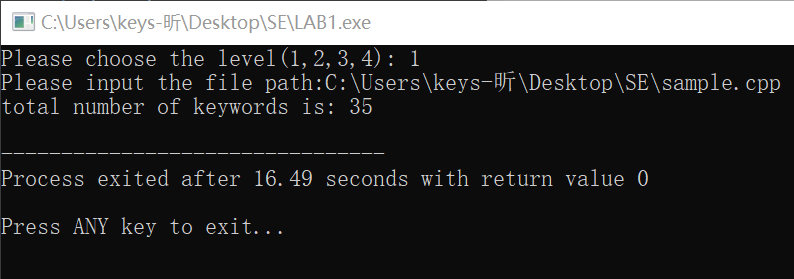### Input level = 2 :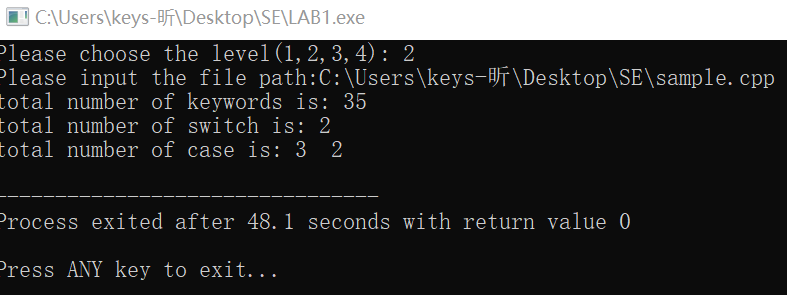### Input level = 3 :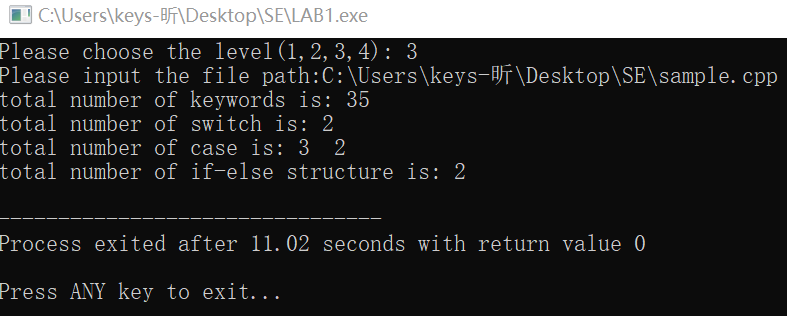### Input level = 4 :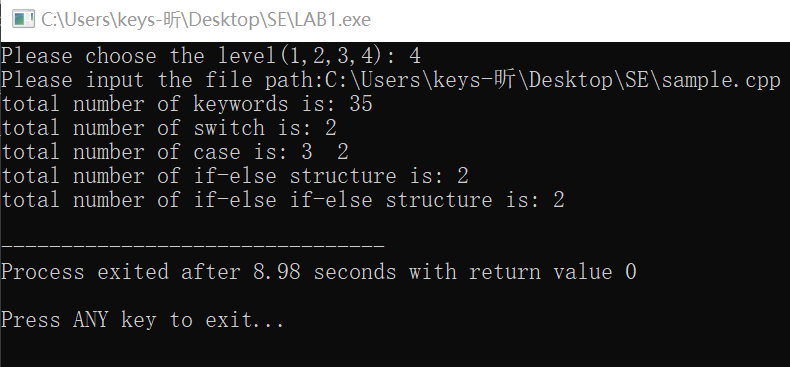# Part6: Coverage optimization & Test performance

## 1.coverage optimization

In unit testing, code coverage is often used as a measure of how good the test is, or even as a measure of how well the test task is done. For example, code coverage must be 80 or 90 percent.

To perform code coverage statistics, simply execute the code being counted through coverage's run parameter. I installed coverage in Vscode via PIP.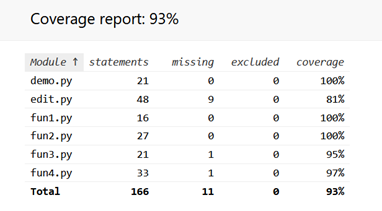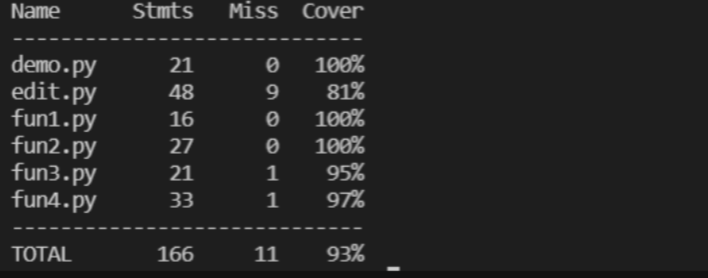The rate of cover is highly as 93% as above, which shows the test is good.

## 2.Test performance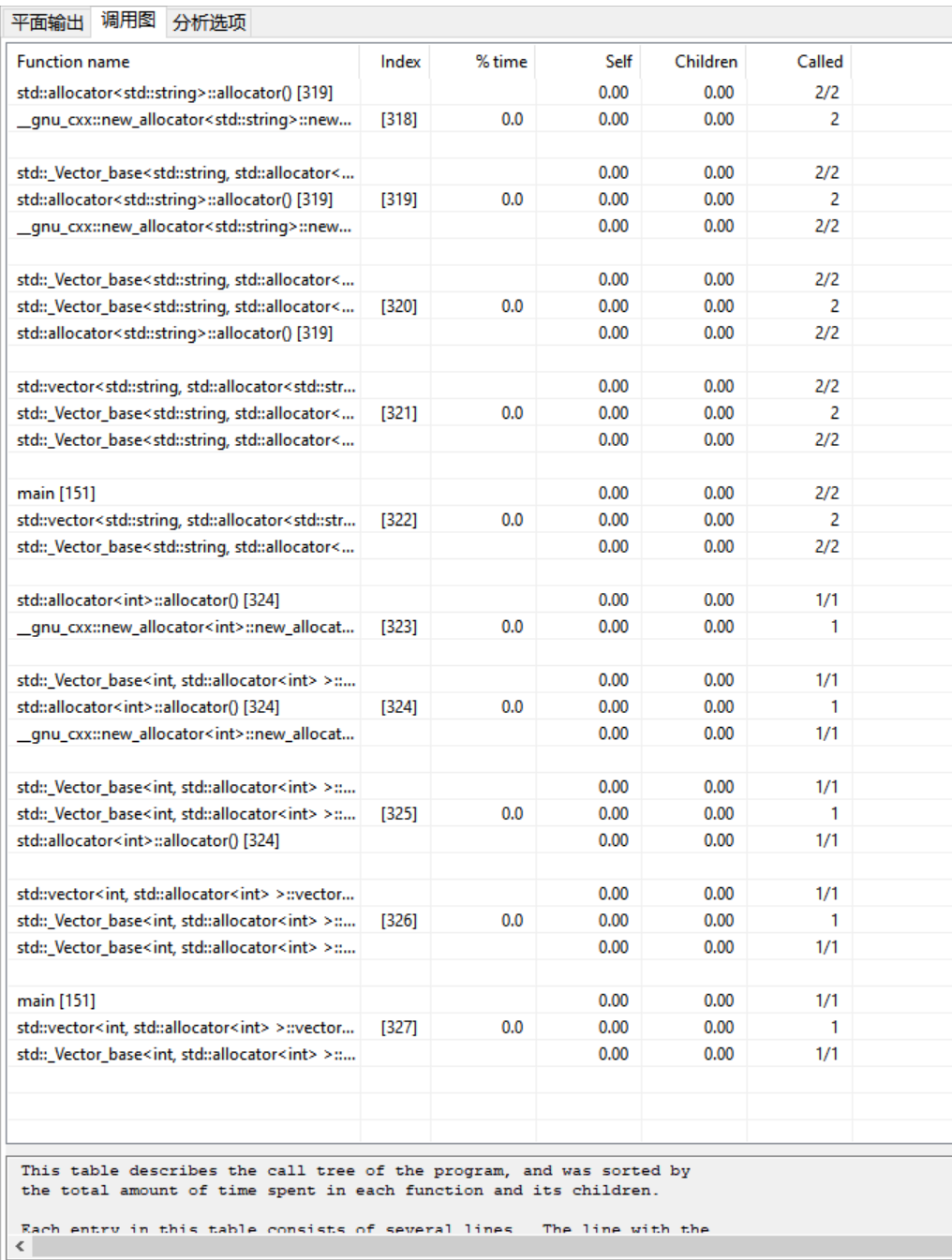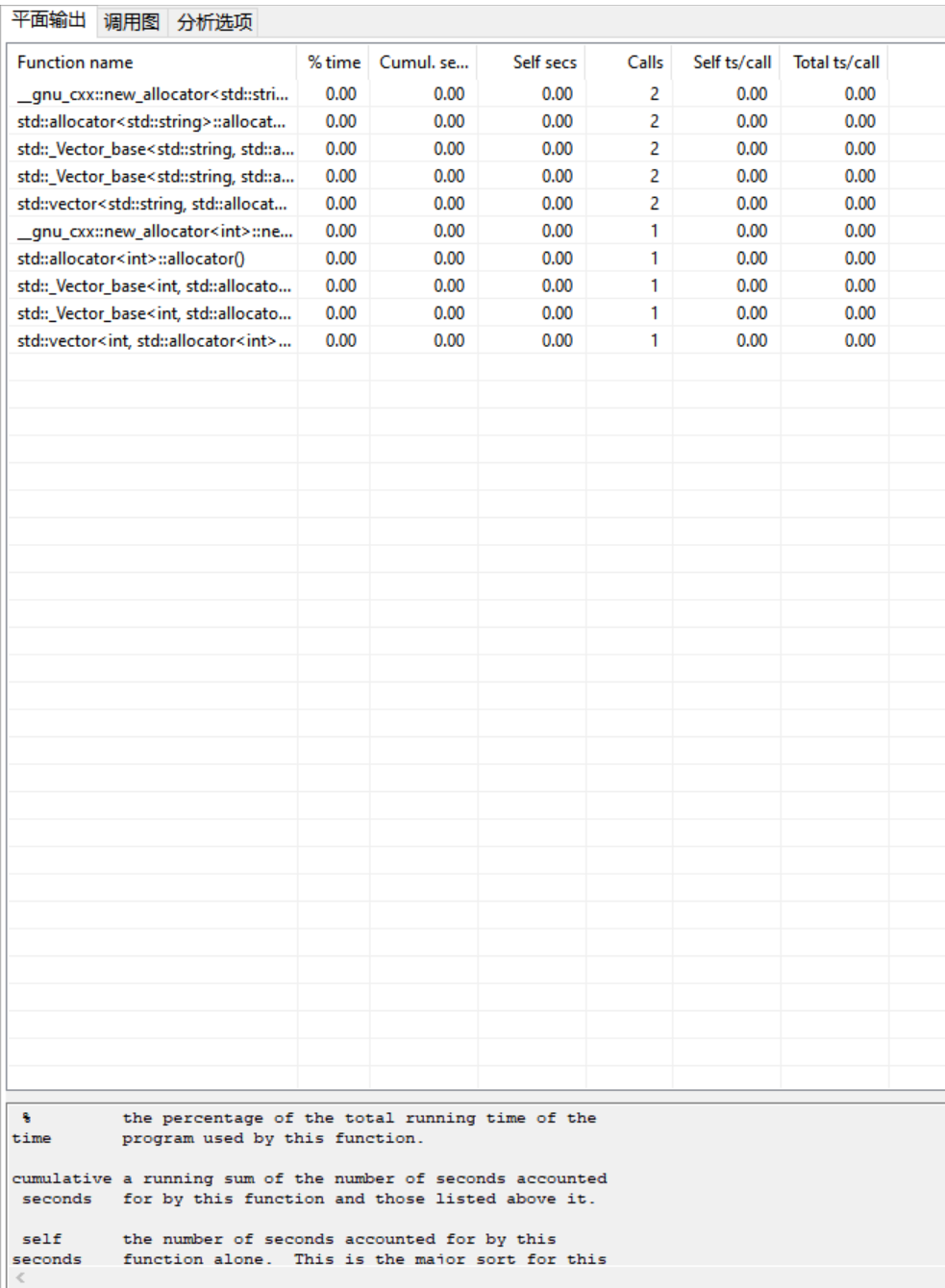# Part7: Summary

For me, this project takes much time enabling me to adjust and adapt to the complete project process. Although I think I should refresh myself in algorithm like iterate the loop and the preprosess of file, through using of the PSP meter also allowed me to better plan my time and keep my tasks running smoothly. After all. I benefited a lot from this Lab.

( a bit tired now..😭😭😭)...全文
164 回复 打赏 收藏 转发到动态 举报SSD6LAB答案的WORD文档EE308FZ Lab_3_1
EE308FZ First BlogEE308FZ Lab3-1】05 group - topic selection and requirement analysis report
This is the Blog for EE308FZ Lab3-1 🚀lab1-2
lab1-2 extract keywordsEE308 Lab1-2
EE308 Lab1-2。

285发帖与我相关我的任务• 近7日
• 近30日
• 至今